﻿ Mechanical System and SimMechanics Simulation

### Mechanical System and SimMechanics Simulation

Darina Hroncova, Miroslav PastorOPEN ACCESSPEER-REVIEWED

## Mechanical System and SimMechanics Simulation

Darina Hroncova1,, Miroslav Pastor1

1Department of applied mechanics and mechatronics, Technical University of Košice / Faculty of mechanical engineering, Košice, Slovakia

### Abstract

The work shows the use of SimMechanics for modeling of mechanical systems. As an example a mechanical model of one degree of freedom is solved by this toolbox for the Matlab/ Simulink environment. This paper describes how to simulate the dynamics of mechanical system with SimMechanics. Mechanical systems are represented by connected block diagrams. Unlike normal Simulink blocks, which represent mathematical operations, or operate on signals, physical modeling blocks represent physical components in SimMechanics, and geometric and kinematic relationships directly. This is not only more intuitive, it also saves the time and effort to derive the equations of motion. SimMechanics models, however, can be interfaced seamlessly with ordinary Simulink block diagrams. This enables the user to design e.g. the mechanical and the control system in one common environment.

### At a glance: Figures

12345
Prev Next

• Hroncova, Darina, and Miroslav Pastor. "Mechanical System and SimMechanics Simulation." American Journal of Mechanical Engineering 1.7 (2013): 251-255.
• Hroncova, D. , & Pastor, M. (2013). Mechanical System and SimMechanics Simulation. American Journal of Mechanical Engineering, 1(7), 251-255.
• Hroncova, Darina, and Miroslav Pastor. "Mechanical System and SimMechanics Simulation." American Journal of Mechanical Engineering 1, no. 7 (2013): 251-255.

 Import into BibTeX Import into EndNote Import into RefMan Import into RefWorks

### 1. Introduction to SimMechanics

As already mentioned, the SimMechanics blocks do not directly model mathematical functions but have a definite physical - mechanical meaning. The block set consists of block libraries for bodies, joints, sensors and actuators, constraints and drivers, and force elements. Besides simple standard blocks there are some blocks with advanced functionality available, which facilitate the modeling of complex systems enormously. An example is the Joint Actuator with event handling for locking and unlocking of the joint. Modeling such a component in traditional ways can become quite difficult. The machine is assembled automatically at the beginning of the simulation .

All blocks are configurable by the user via graphical user interfaces as known from Simulink. The option to generate or change models from Matlab programs with certain commands is not implemented yet. It might be added in future releases. It is possible to extend the block library with custom blocks, if a problem is not solvable with the provided blocks. These custom blocks can contain other preconfigured blocks or standard Simulink S-functions.

Standard Simulink blocks have distinct input and output ports. The connections between those blocks are called signal lines, and represent inputs to and outputs from the mathematical functions. Due to Newton’s third law of action and reaction, this concept is not sensible for mechanical systems. If a body A acts on a body B with a force F, B also acts on A with a force −F, so that there is no definite direction of the signal flow. Special connection lines, anchored at both ends to a connector port have been introduced with this toolbox. Unlike signal lines, they cannot be branched, nor can they be connected to standard blocks. To do the latter, SimMechanics provides Sensor and Actuator blocks. They are the interface to standard Simulink models .

Actuator blocks transform input signals in motions, forces or torques. Sensor blocks do the opposite, they transform mechanical variables into signals.

### 2. The Mechanism Example

Some of the features of SimMechanics will now be shown on an example. Figure 1 shows the mechanical system of interest. It is mechanical system with one degree of freedom. It consists of a mass m attached to a ground which slides on a smooth horizontal plane.

Mass m is attached to ground with a linear spring/ damper system with the spring stiffness k and the damping coefficient b. The system has one translational degree of freedom, q1 = x. The equations of motion for that mechanism can be derived by Hamilton’s, Lagrange equations or Newton’s second law.

2.1. Formulation of the Equation of Motion

Equation of the motion of the mechanical system in axis x [1, 2, 3]: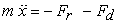(1)

Where: Fr , Fd – reaction forces in the springs and damping forces of system.

Reaction forces in the springs and damping forces are: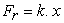(2)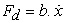(3)

Equation of the motion of the mechanical system: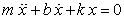(4)

We obtain equations of motion of the form :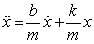(5)

The aim is to determine the response of the oscillating system - displacements x=x(t). This, however, requires solve a system of differential equations. For a mechanical system with one degree of freedom it is a homogeneous system of 2nd order linear differential equation with constant coefficients.

The block diagram in Simulink from equation of motion (5) mechanical system:

Block diagram in next form:

M-file for graphical results x(t), v(t) and a(t):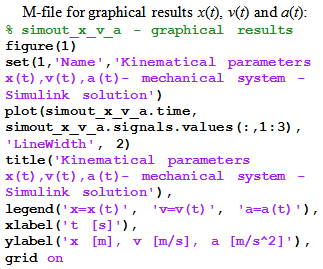Export Setup window with Properties:

The widow in Simulink Figure 1 with result parameters in graphical form:

M-file for result x(t) in graphic form [4, 5]: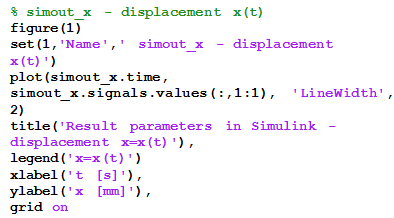Result parameter of the velocity in the form: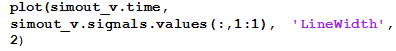Result parameter of the velocity in the form: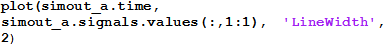### 4. SimMechanics Solution

The Physical Modeling environment SimMechanics it makes the task easy . Simulink block diagram shown in Figure 11 describe model of the mechanism without writing any equations [8, 9]. Let us have a closer look at the diagram. Obviously, every block corresponds to one mechanical component. The properties of the blocks can be entered by double-clicking on them. These are for example mass properties, dimensions and orientations for the bodies, the axis of rotation for the rotational joint and the spring/ damper coefficients for the Spring & Damper block. The initial conditions are given directly by specifying the initial position and orientations of the rigid bodies.

Model of the mechanism is so simple, it lacks some important features, though. It is not possible to specify nonzero initial conditions for velocities and accelerations, and no data is output to the workspace for further processing. This can be done by adding Sensor blocks and Joint Initial Condition block . Model which is functionally completely equivalent to the one in Figure 11 is build. It is shown in Figure 11. The Joint Initial Condition blocks in SimMechanics let the users define arbitrary initial conditions, and the Joint Sensor blocks measure the position, velocity, and acceleration of the two independent motion variables. If desired, the forces and torques transmitted by the joints can be sensed, too. Remarkable are the different types of connectors between the blocks. It is clearly visible that the input of the Sensor blocks are the special SimMechanics connection lines, while the output are normal Simulink signals. The signal from SimMechanics can be conducted to the Simulink workspace.

Block Parameters for Body_1:

SimMechanics is an interesting and important add-on to the simulation environment Simulink . It allows to easily include mechanical subsystems into Simulink models, without the need to derive the equations of motion. Especially non-experts will benefit from the visualization tools, as they facilitate the modeling and the interpretation of results. SimMechanics is certainly not a replacement for specialized multibody dynamics software packages due to the limitations of the physical modeling approach and the restrictions of the Simulink environment. It is, however, well suited for many practical problems in education and industry, where Matlab became the standard language of technical computing.

### 5. Conclusion

The paper describes the compilation of the equations of motion of a mechanical system with one degree of freedom in Matlab/Simulink and by SimMechanics.

The results of all models are of course identical. Figure 8, Figure 9 and Figure 10 shows the result of the simulation. Results are shown graphically. The calculation is done for a model in Matlab/Simulink, to illustrate the methodology and in SimMechanics.

In this paper, the Matlab toolbox SimMechanics was introduced, it basic functionalities were shown on an example.

The contribution of this work is primarily educational, especially in the field of Applied Mechanics and Mechatronics. The above procedure presents the possibility of practical implementation of this solution to simple equations of motion of a mechanical system in Matlab/Simulink and SimMechanics.

### Acknowledgement

This work was supported by grant project VEGA No. 1/1205/12 and by project "Research modules for intelligent robotic systems " (IMTS: 26220220141), on the basis of Operational Programme Research and Development financed by European Regional Development Fund.

### References

  Brepta, R., Púst, L., Turek, F.: Mechanické kmitání, Sobotáles, Praha, 1994.In article  Grepl, R., Modelování mechatronických systémů v Matlab SimMechanics. Praha, 2007.In article PubMed  Juliš, K., BREPTA, R., Mechanika II.díl, Dynamika, SNTL, Praha, 1987.In article  Karban, P., Výpočty a simulace v programech Matlab a Simulink, Computer Press, Brno, 2006.In article  Kozák, Š., KAJAN, S., Matlab - Simulink I, STU, Bratislava, 2006.In article  Schlotter, M., Multibody System Simulation with SimMechanics.In article  Záhorec, O., Caban, S., Dynamika, Olymp, Košice, 2002.In article PubMed  Virgala, , Frankovský, P., Kenderová, M., Friction Effect Analysis of a DC Motor. In: American Journal of Mechanical Engineering. 2013. Vol. 1, no. 1 (2013), p. 1-5.In article  Vittek, J., Matlab pre elektrické pohony, Žilina, 1997, 83 p.In article